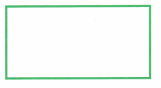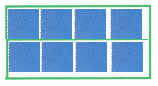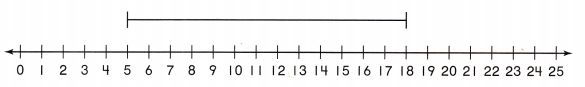# Texas Go Math Grade 2 Module 17 Assessment Answer Key

Refer to our Texas Go Math Grade 2 Answer Key Pdf to score good marks in the exams. Test yourself by practicing the problems from Texas Go Math Grade 2 Module 17 Assessment Answer Key.

## Texas Go Math Grade 2 Module 17 Assessment Answer Key

Concepts and Skills

Use color tiles to cover the rectangle.
Trace around the square tiles. Write how many. TEKS 2.9.F

Question 1.Number of rows: ___
Number of columns: ___
Total: ___ square unitsExplanation:
Number of rows: 2
Number of columns: 4
Total: 8 square units

Measure the length to the nearest centimeter. TEKS 2.9.D

Question 2.___ centimetersExplanation:
The length of the ribbon measures 13 centimeters.
placed the left edge of the object with the zero mark on the ruler.

Measure the real object to the nearest centimeter. Then measure to the nearest meter. TEKS2.9.B,2.9.D

Question 3.
bulletin board___ centimeters
___ meters
200 centimeters
20 meters
Explanation:
1 meter is equal to 100 centimeters

Texas Test Prep

Fill in the bubble for the correct answer choice.

Question 4.
Use a centimeter ruler. Which is the best choice for the length of the color pencil? TEKS 2.9.D(A) 8 centimeters
(B) 10 centimeters
(C) 5 centimetersExplanation:
The length of the pencil measures 8 centimeters.
placed the left edge of the object with the zero mark on the ruler.

Question 5.
Use a centimeter ruler. Which is the best choice for the length of the toothpick TEKS 2.9.D(A) 10 centimeters
(B) 5 centimeters
(C) 7 centimetersExplanation:
The length of the Tooth pick measures 5 centimeters.
placed the left edge of the object with the zero mark on the ruler.

Question 6.
Joshua has a rope that he measures in centimeters. He draws the diagram below to show the length of the rope.
How many centimeters long is his rope? TEKS 2.9.C(A) 13 centimeters
(B) 18 centimeters
(C) 5 centimeters